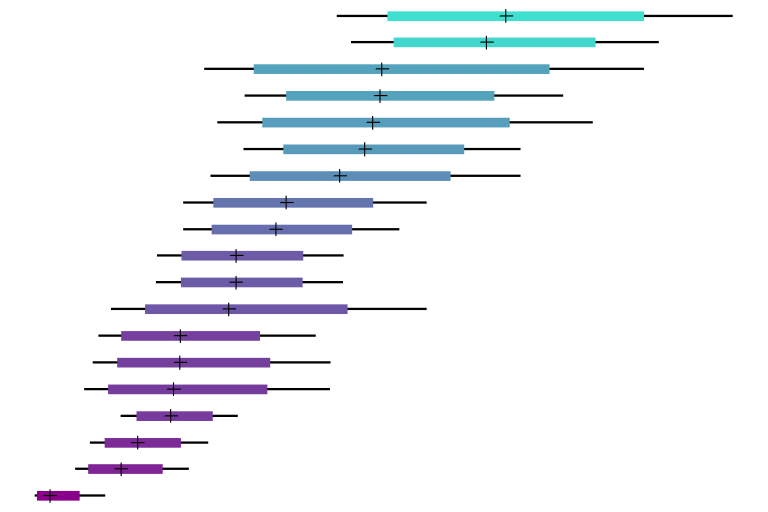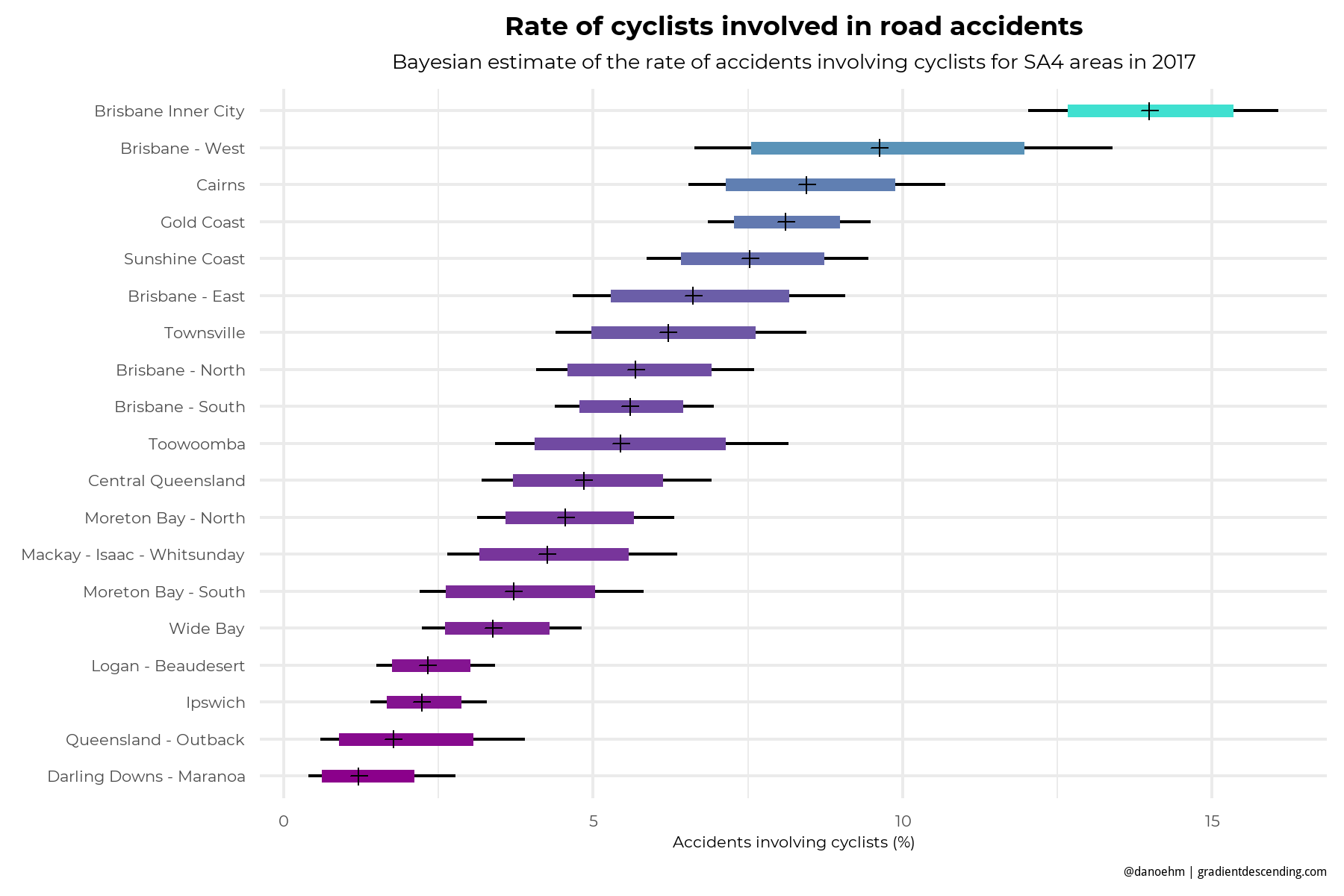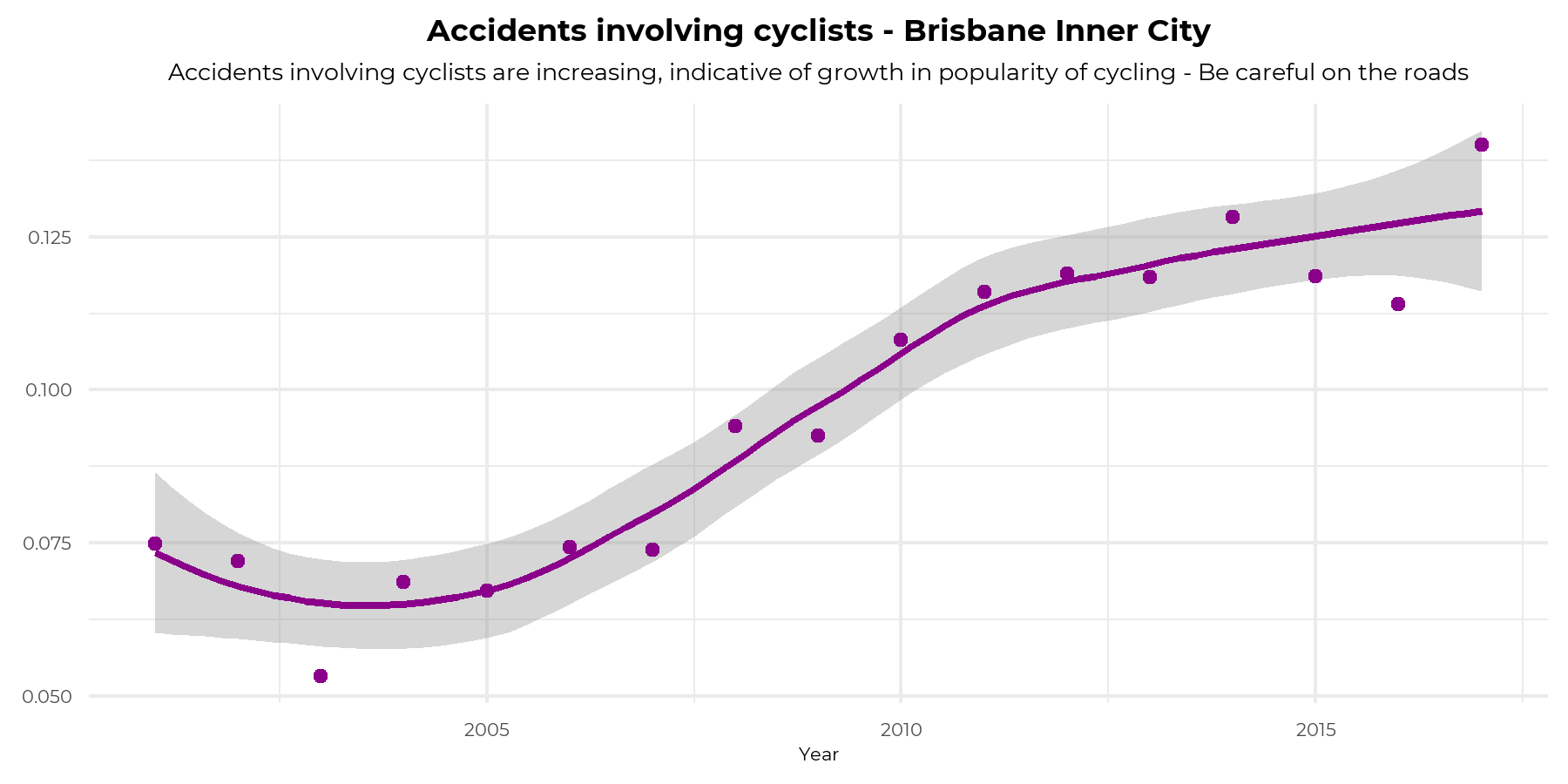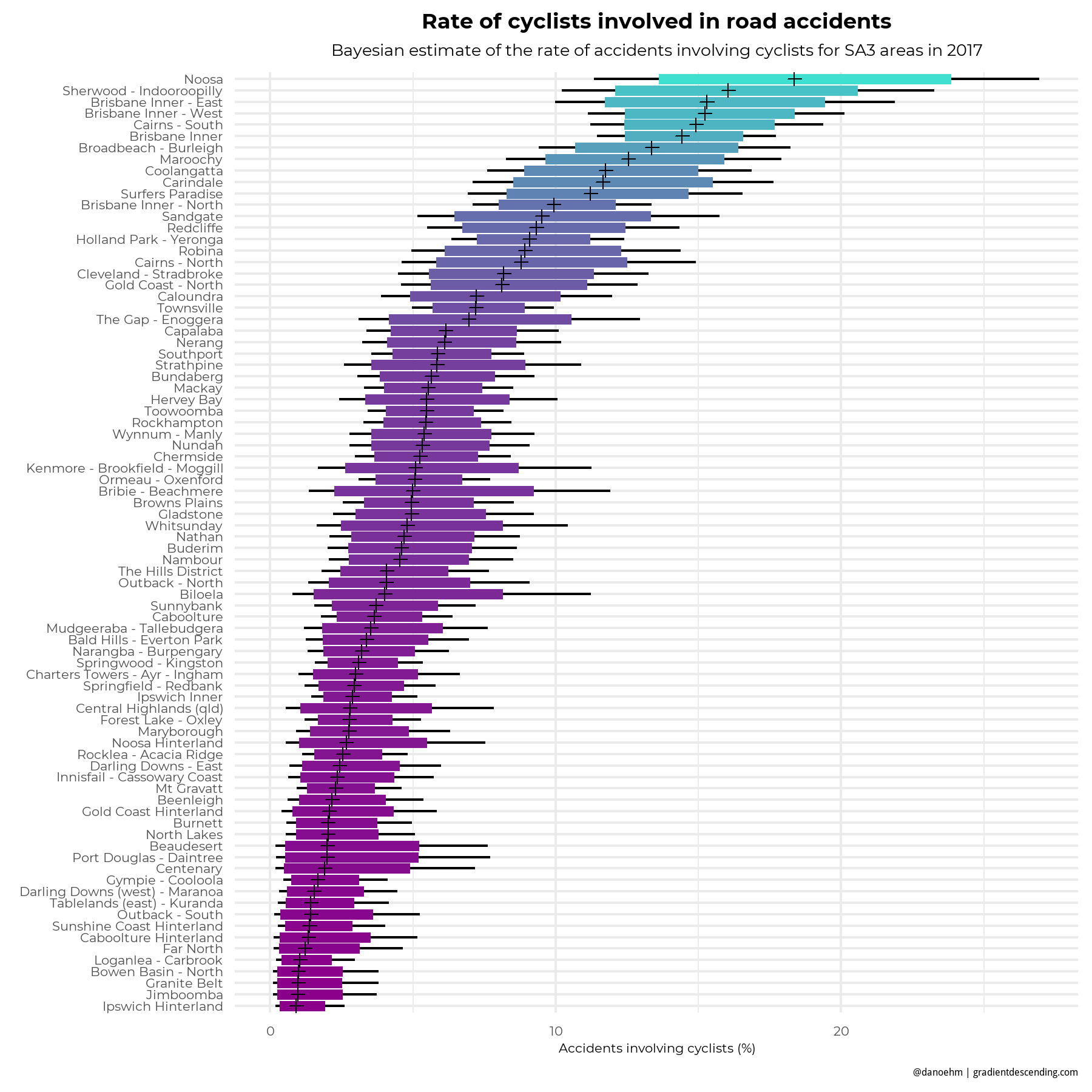Want to share your content on R-bloggers? click here if you have a blog, or here if you don't.In my previous post I built a Shiny app mapping accidents on Queensland roads which was great at showing the problematic areas within cities and regional areas. I have added to this by estimating the fatality rate given the observed accidents and the rate of accidents involving cyclists for SA3 and SA4 areas. I have also updated the filters making them tidier. What follows in commentary of what can be found in the Shiny app. If you want to jump straight to it, run

shiny::runGitHub("doehm/road-accidents/", "doehm", launch.browser = TRUE)

## Most dangerous areas

I used a Bayesian approach to estimate the fatality rate for 2017 (the data isn’t complete for 2018) and presented it as a proportion of the number of observed road accidents. The data dates back to 2001 but it’s reasonable to use the most recent data to allow for improvements in road conditions, policies, advertising, population growth, etc which may have an underlying impact on the risk of road accidents.

To construct a prior I used years 2014-2016 at SA3 level. By taking 10,000 draws from the posterior we estimate the fatality rate distributions for each area. The bands represent the 80% and 95% prediction interval.

The top 3 most dangerous SA4’s for 2017 are Mackay – Isaac – Whitsunday, Wide Bay and The Queensland outback. It’s not surprising that the large majority of fatalities occurred at high speeds on highways and back roads. Having said that a few have occurred closer to the town centers in these areas. It’s possible the road conditions are not particularly good but that’s hard to determine. The road condition filter only has 4 states, sealed (dry / wet) and unsealed (dry / wet) and doesn’t offer much more information. It appears dry conditions on sealed roads is when most accidents occur.

The 3 least dangerous areas are Brisbane North, Brisbane Inner City and Brisbane South. Again, it shouldn’t be surprising given the speed limit is lower in the city, if accidents occur they are generally less severe. There are a lot of rear ended accidents and accidents when leaving a car park – the classic.At the SA3 level the incident rates focus on smaller geographical areas which can highlight other features. For example, at the SA4 level the area often includes the city centers and the Hinterland regions which tend to have different rates where it’s often higher in the Hinterlands. This is largely due to the differing speed limits. The most dangerous areas are Burnett, Port Douglas and the Ipswich Hinterlands. The least dangerous are Chermside, Brisbane Inner West and Springfield – Redbank. Click the image to zoom in, there are a lot of SA3’s.## Most dangerous areas for cyclists

As a cyclist it’s good to know which roads to pay particular attention on. In a similar way we’ll look at the rate of road accidents involving cyclists by area. An influencing factor is how many cyclists are on the roads relative to cars and other vehicles. If the area has a strong cycling culture it’s more likely that an accident will involve a cyclist.It’s not surprising the dense city centers with more traffic have more accidents involving cyclists. At the SA4 level it’s pretty clear that Brisbane Inner City is a dangerous place to ride a bike, particularly on Adelaide street which you can see from the app (go to show filter > unit type involved > bicycle). The rate of accidents involving cyclists in Brisbane Inner City is significantly higher than all other SA4’s. I’m curious to know if the the growth of the CityCycle scheme (which is awesome by the way) is somewhat a contributing factor. Although, given this was introduced in 2011, and 2005-2010 saw the highest rate of growth in accidents involving cyclists, probably isn’t the biggest factor in the high rate of accidents but it should contribute if more people are on bikes – they’re also not the easiest bikes in the world to ride. The other areas in the top 3, Brisbane City West and Cairns. Sheridan Street, the main drag in Cairns is where you need to be extra vigilant.Here Noosa tops the chart for 2017. It sits within the Sunshine Coast SA4 region ranking 5th in the chart above which also includes the Hinterland regions which is why it scores lower above. Noosa has a strong cycling culture which could be influencing it’s high rate. With a median of a whopping 18% definitely keep your eyes peeled when cycling on those roads. Also, take care in Sherwood – Indooroopilly. Keep in mind that these areas are not necessarily where the most accidents involving cyclists occur. Rather if there is an accident it’s more likely to have involved a cyclists relative to other areas. If you’re driving in those areas keep an eye out for cyclists on the road, perhaps there aren’t marked cycling lanes or off-road bike paths or some dodgy intersections.Ideally the rate would be adjusted for the number of cyclists on the road, however this is still a useful statistic to find the more dangerous areas. This analysis has also been done for pedestrians involved in car accidents which is can be found in the app. Just a heads up, be careful in Fortitude Valley.

## Code bits

Run the shiny app

library(shiny)
runGitHub("doehm/road-accidents/", "doehm", launch.browser = TRUE)

library(repmis)
source_data("https://github.com/doehm/road-accidents/raw/master/data/road-accident-data.Rdata")

Estimate hyperparameters

# get prior information from previous 3 years
# use method of moments to get alpha and beta
# ideally we would use a hierarchical model but this is suitable
hyper_params <- function(y){
mu <- mean(y)
s2 <- var(y)
gamma <- mu*(1-mu)/s2-1
alpha <- gamma*mu
beta <- gamma*(1-mu)
return(list(alpha = alpha, beta = beta))
}

# estimating the actual parameters
df <- accidents_raw %>%
filter(
Loc_Queensland_Transport_Region != "Unknown",
Crash_Year >= 2014,
Crash_Year <= 2016
) %>%
group_by(Loc_ABS_Statistical_Area_3) %>%
summarise(
count = n(),
n_fatalities = sum(Count_Casualty_Fatality)
) %>%
mutate(theta = n_fatalities/(count))

hyper_params(df$theta) Forest plots library(tidyverse) library(showtext) # font font_add_google(name = "Montserrat", family = "mont") showtext_auto() # SA3 forest plot of fatality rate # set my theme and colours theme_forest <- function(scale = 1){ theme_minimal() + theme( legend.position = "none", axis.text = element_text(family = "mont", size = 16*scale), axis.text.x = element_text(vjust = 0.5), axis.title.y = element_blank(), axis.title.x = element_text(family = "mont", size = 16*scale), plot.title = element_text(family = "mont", hjust = 0.5, size = 26*scale, face = "bold"), plot.subtitle = element_text(family = "mont", hjust = 0.5, size = 20*scale), plot.caption = element_text(size = 12*scale) ) } my_cols <- function(n = 16) colorRampPalette(c("darkmagenta", "turquoise"))(n) # simulate from the posterior posterior_f <- function(df, y, n, a = 1.3, b = 77, inflator = 100) { out <- data.frame() qs <- c(0.025, 0.1, 0.5, 0.9, 0.975) for(k in 1:nrow(df)){ out <- rbind(out, inflator*rgamma(1e4, shape = a+y[k], rate = b+n[k]) %>% quantile(qs)) } colnames(out) <- paste0("q", 100*qs) return(out) } # SA4 # fatalities areas <- grep("Area", colnames(accidents_raw), value = TRUE)[3:4] names(areas) <- c("sa3", "sa4") fatality_fn <- function(area){ accidents_raw %>% group_by_(area) %>% filter( Crash_Year == 2017, ) %>% summarise( count = length(Count_Casualty_Total), n_fatalities = sum(Count_Casualty_Fatality) ) %>% bind_cols(posterior_f(df = ., y = .$n_fatalities, n = .$count)) %>% arrange(q50) %>% mutate_(area = interp(~factor(v, level = v), v = as.name(area))) %>% ggplot() + geom_segment(mapping = aes(x = q2.5, xend = q97.5, y = area, yend = area)) + geom_segment(mapping = aes(x = q10, xend = q90, y = area, yend = area, col = q50), size = 2) + geom_point(mapping = aes(x = q50, y = area), pch = 3) + theme_forest() + scale_colour_gradientn(colors = my_cols()) + labs( title = "Fatality rate given observed road accidents", subtitle = paste("Bayesian estimate of the fatality rate for", toupper(names(area)), "areas in 2017"), x = "Fatality rate (%)") } fatality_plots <- list(sa3 = fatality_fn(areas), sa4 = fatality_fn(areas)) Cyclist plot # cyclists df <- accidents_raw %>% filter( Crash_Year >= 2014, Crash_Year <= 2016, Loc_ABS_Statistical_Area_3 != "Unknown" ) %>% group_by(Loc_ABS_Statistical_Area_3) %>% summarise( n_bicycles = sum(Count_Unit_Bicycle > 0), n_accidents = n() ) %>% mutate(p = n_bicycles/n_accidents) %>% arrange(desc(p)) # estimate hyperparameters hyper_params(df$p)

# cyclists
cyclist_fn <- function(area){
accidents_raw %>%
group_by_(area) %>%
filter(Crash_Year == 2017) %>%
summarise(
count = n(),
n_bicycles = sum(Count_Unit_Bicycle > 0)
) %>%
bind_cols(posterior_f(df = ., y = .$n_bicycles, n = .$count, a = 1.55, b = 25)) %>%
arrange(q50) %>%
mutate_(area = interp(~factor(v, level = v), v = as.name(area))) %>%
ggplot() +
geom_segment(mapping = aes(x = q2.5, xend = q97.5, y = area, yend = area)) +
geom_segment(mapping = aes(x = q10, xend = q90, y = area, yend = area, col = q50), size = 2) +
geom_point(mapping = aes(x = q50, y = area), pch = 3) +
theme_forest() +
labs(
title = "Rate of cyclists involved in road accidents",
subtitle = paste("Bayesian estimate of the rate of accidents involving cyclists for", toupper(names(area)), "areas in 2017"),
x = "Accidents involving cyclists (%)"
)
}
cyclist_plots <- list(sa3 = cyclist_fn(areas), sa4 = cyclist_fn(areas))

# Brisbane inner
accidents_raw %>%
filter(Loc_ABS_Statistical_Area_4 == "Brisbane Inner City", Crash_Year < 2018) %>%
group_by(Crash_Year) %>%
summarise(
n_bikes = sum(Count_Unit_Bicycle > 0),
n_accidents = n(),
p_bikes = n_bikes/n_accidents
) %>%
bind_cols(posterior_f(df = ., y = .$n_bikes, n = .$n_accidents, a = 1.55, b = 25)/100) %>%
ggplot(aes(x = Crash_Year, y = q50)) +
geom_line(col = "darkmagenta") +
geom_point(col = "darkmagenta") +
theme_minimal() +
theme_forest() +
labs(
x = "Year",
title = "Accidents involving cyclists - Brisbane Inner City",
subtitle = "Accidents involving cyclists are increasing, indicative of growth in popularity of cycling - Be careful on the roads"
)